Next: Filter estimation Up: Estimation of nonstationary PEFs Previous: Estimation of nonstationary PEFs

# Definitions

I call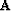the convolution or combination operator with a bank of non-stationary filters. For the non-stationary convolution, the filters are in the column of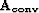(one filter corresponds to one point in the input space) whereas for the non-stationary combination, the filters lie in the rows of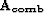(one filter corresponds to one point in the output space). For the convolution matrix, I define ai,j as the ith coefficient of the filter for the jth data point in the input space. For the combination matrix, I define ai,j as the jth coefficient of the filter for the ith data point in the output space. Therefore, for the non-stationary convolution we have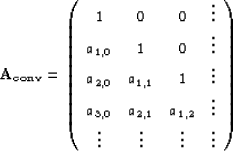(120)
and for the non-stationary combination we have(121)
The size of both matrices is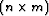where n is the size of an output vector () and m the size of an input vector () if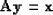(122)
The helical boundary conditions allow to generalize this 1-D convolution to higher dimensions. We can rewrite equation () for the convolution as follows:(123)
where nf is the number of filter coefficients, and for the combination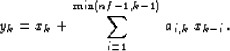(124)
In the next section, I show how the non-stationary PEFs are estimated.Next: Filter estimation Up: Estimation of nonstationary PEFs Previous: Estimation of nonstationary PEFs
Stanford Exploration Project
5/5/2005